# scipy.spatial.distance.hamming¶

scipy.spatial.distance.hamming(u, v)

Computes the Hamming distance between two n-vectors u and v, which is simply the proportion of disagreeing components in u and v. If u and v are boolean vectors, the Hamming distance is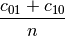where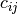is the number of occurrences of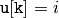and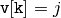for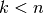.

Parameters : u : ndarray An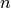-dimensional vector. v : ndarray An-dimensional vector. d : double The Hamming distance between vectors u and v.

#### Previous topic

scipy.spatial.distance.euclidean

#### Next topic

scipy.spatial.distance.jaccard